# Online Electronics and Communication Engineering Test - Communication Systems Test 2Loading Test...

Instruction:

• This is a FREE online test. DO NOT pay money to anyone to attend this test.
• Total number of questions : 20.
• Time alloted : 30 minutes.
• Each question carry 1 mark, no negative marks.
• DO NOT refresh the page.
• All the best :-).

1.

A tweeter should be fed from the input through a

A.
 low pass filterB.
 high pass filterC.
 band pass filterD.
 band stop filterExplanation:

Tweeter is high frequency loudspeaker covering the range above 5000 Hz.

2.

In the equivalent circuit of a moving coil cone type loudspeaker, the mass of the moving system is represented by

A.
 a resistanceB.
 a capacitanceC.
 an inductanceD.
 a conductanceExplanation:

Mass has inertia and is analogous to inductance.

3.

A directional microphone gives an output of 0.5 mV whereas an omnidirectional one gives an output of 0.5 μV in the same position for the same sound pressure. The directivity of the directional microphone is

A.
 120 dBB.
 90 dBC.
 60 dBD.
 30 dBExplanation:

Directivity =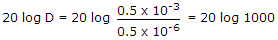= 60 dB.

4.

The most commonly used transistor amplifier circuit is

A.
 CBB.
 CCC.
 CED.
 CE or CCExplanation:

Common emitter connection has high power gain, and good current and voltage gains.

5.

Full duplex operation

A.
 requires modems at both ends of circuitB.
 requires two pairs of cablesC.
 can transfer data in both directions simultaneouslyD.
 all of the aboveExplanation:

Full duplex system has all these features.

6.

Consider the following statements as regards DPSK and DEPSK

1. DPSK demodulator requires a device which operates at carrier frequency and provides a delay of bit time Tb
2. In DPSK, synchronous demodulation recovers the signal b(t) and the decoding of b(t) to generate d(t) is done at base band
3. In both DPSK and DEPSK errors always occur in pairs
Which of the above statements are correct?

A.
 1 and 3B.
 1 and 2C.
 2 and 3D.
 1, 2 and 37.

Consider the following statements about PCM

1. PCM is not noise resistant
2. PCM requires complex encoding and quantizing circuitry
3. PCM requires large bandwidth
Which of the above are correct?

A.
 1 onlyB.
 1, 2, 3C.
 2, 3D.
 1 and 38.

In a TV studio the illuminance is about

A.
 200 luxB.
 1000 luxC.
 10000 luxD.
 10 lux9.

Bandwidth for practical PCM system is (where n is no. of channels, N length of PCM codes)

A.
 2n N fm HzB.
 4n fm HzC.
 2n fmD.
 (N + 1)fm10.

In radar PRF depends on maximum range.

A.
 TrueB.
 False11.

A.
 the highest AGC voltage is produced between stationsB.
 an increase in signal strength produces more AGCC.
 the audio stage gain is normally controlled by AGCD.
 the faster the time constant of AGC, more accurate the output12.

If B is channel bandwidth, S is signal power and N is the total noise within the channel bandwidth, the channel capacity C of a white band limited gaussian channel is

A.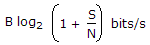B.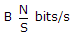C.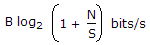D.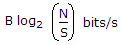13.

Industrial noise has the frequency range of

A.
 1 to 100 HzB.
 200 to 5000 HzC.
 above 200 MHzD.
 1 to 600 MHz14.

In phase modulation, the phase deviation is

A.
 proportional to the amplitude of the signal and varies with frequencyB.
 remains constant while amplitude and frequency of signal variesC.
 proportional to the amplitude of the signal and independent of the frequencyD.
 none of the above15.

BFO stands for

A.
 Beat Frequency OscillatorB.
 Broad Frequency OscillatorC.
 Blocking Frequency OscillatorD.
 Ballistic Frequency Oscillator16.

A lens has a hyperfocal distance of 7.5 m. It is focused at 7.5 m. Then

A.
 all objects from 7.5 m to infinity will appear in focusB.
 all objects from 3.75 m to infinity will appear in focusC.
 all objects from 0 to 7.5 m will appear in focusD.
 all objects from 0 to 3.75 m will appear in focus17.

The highest modulating frequency typically used in AM systems is

A.
 1 kHzB.
 5 kHzC.
 20 kHzD.
 50 kHz18.

The number of spectral component of impulse wave is

A.
 1B.
 0C.
 2D.
 ∞19.

In amplitude modulation

A.
 the amplitude of carrier varies in accordance with the amplitude of the modulating signal.B.
 the modulating frequency lies in the audio rangeC.
 the amplitude of the carrier remains constantD.
 the amplitude of the carrier varies in accordance with the frequency of the modulating signal.20.

If S represents the carrier synchronization at the receiver and r represents the bandwidth efficiency, then the correct statement for the coherent binary PSK is

A.
 r = 0.5, S is requiredB.
 r = 1.0, S is requiredC.
 r = 0.5, S is not requiredD.
 r = 1.0, S is not required